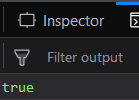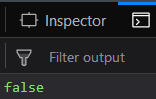# Underscore.js _.isArray() Function

• Last Updated : 25 Nov, 2021

The Underscore.js is a JavaScript library that provides a lot of useful functions like the map, filter, invoke etc even without using any built-in objects.

The _.isArray() function is used to find whether the passed argument is an array or not. An array is a set of variables, constants, special symbols. True will be returned if the object is an array else false will be returned. The array can have different names and can be of any size even zero.

Syntax:

`_.isArray(object)`

Parameters:
It takes only one argument, i.e., the object that needs to be checked.

Return value:
It returns ‘true’ if the argument passed si an array else it returns ‘false’.

1. Passing an array of 3 numbers to the _.isArray() function:
The _.isArray() function takes the element passed and checks whether it is an array or not. Since the passed argument is a set of 3 numbers – 1, 2, 3. Therefore, it is an array. And Hence, the final output will be true.
Examples:
 `<``html``>`` ` `<``head``>``    ``<``script` `src``=``"https://cdnjs.cloudflare.com/ajax/libs/underscore.js/1.9.1/underscore-min.js"``>``  `````` ` `<``body``>``    ``<``script` `type``=``"text/javascript"``>``        ``console.log(_.isArray([1, 2, 3]));``    `````` ` ``

Output:2. Passing an array of characters to the _.isArray() function:
The argument passed to the _.isArray() function will be checked that is it an array or not. Since the argument is containing only one character, “a” but is inside [] brackets so it is an array. And hence the answer is true.
Example:
 `<``html``>`` ` `<``head``>``    ``<``script` `src``=``"https://cdnjs.cloudflare.com/ajax/libs/underscore.js/1.9.1/underscore-min.js"``>``  `````` ` `<``body``>``    ``<``script` `type``=``"text/javascript"``>``        ``console.log(_.isArray(["a"]));``    `````` ` ``

Output:3. Passing an empty array to _.isArray() function:
The _.isArray() function takes the element which here is [] and then checks whether it is an array or not. Since there is not element inside the [] brackets so, it is empty. But since [] brackets are present so it is an array. Since empty array is also an array so the output is true.
Example:
 `<``html``>`` ` `<``head``>``    ``<``script` `src``=``"https://cdnjs.cloudflare.com/ajax/libs/underscore.js/1.9.1/underscore-min.js"``>``  `````` ` `<``body``>``    ``<``script` `type``=``"text/javascript"``>``        ``console.log(_.isArray([]));``    `````` ` ``

Output:4. Passing a number to the _.isArray() function:
If we pass any random number to the _.isArray() function then as it will check that the argument is an array or not. Since, a number is passed to the _.isArray() function therefore the output will be false.
Example:
 `<``html``>`` ` `<``head``>``    ``<``script` `src``=``"https://cdnjs.cloudflare.com/ajax/libs/underscore.js/1.9.1/underscore-min.js"``>``  `````` ` `<``body``>``    ``<``script` `type``=``"text/javascript"``>``        ``console.log(_.isArray(1));``    `````` ` ``

Output:`

NOTE:
These commands will not work in Google console or in firefox as for these additional files need to be added which they didn’t have added.
 `<``script` `type``=``"text/javascript"` `src` `=``"https://cdnjs.cloudflare.com/ajax/libs/underscore.js/1.9.1/underscore-min.js"``>```